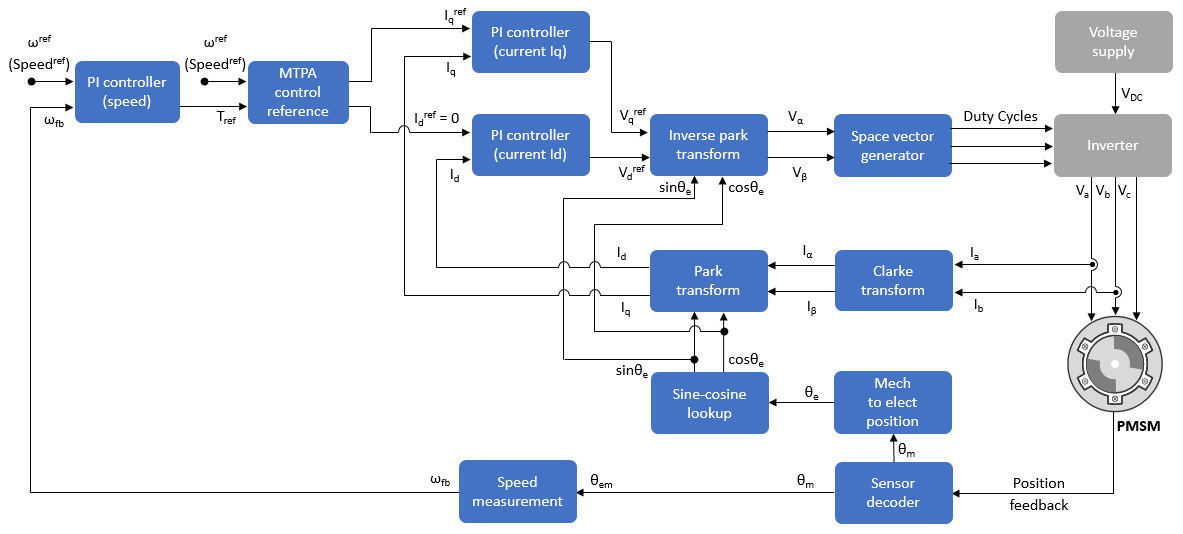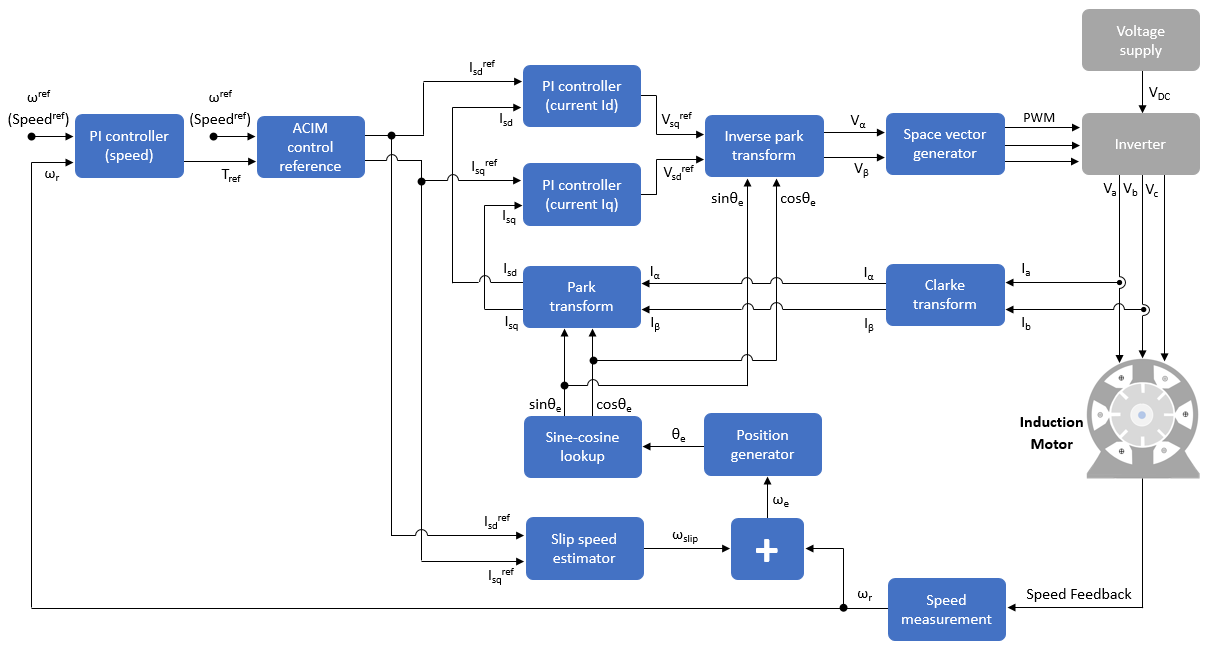## Field-Oriented Control (FOC)

Field-Oriented Control (FOC), also known as vector control, is a technique used to control Permanent Magnet Synchronous Motor (PMSM) and AC induction motors (ACIM). FOC provides good control capability over the full torque and speed ranges. The FOC implementation requires transformation of stator currents from the stationary reference frame to the rotor flux reference frame (also known as d-q reference frame).

Speed control and torque control are the most commonly used control modes of FOC. The position control mode is less common. Most of the traction applications use the torque control mode in which the motor control system follows a reference torque value. In the speed control mode, the motor controller follows a reference speed value and generates a torque reference for the torque control that forms an inner subsystem. In the position control mode, the speed controller forms the inner subsystem.

FOC algorithm implementation requires real time feedback of the currents and rotor position. Measure the current and position by using sensors. You can also use sensorless techniques that use the estimated feedback values instead of the actual sensor-based measurements.

### Permanent Magnet Synchronous Motor (PMSM)

This figure shows the FOC architecture for a PMSM. For detailed set of equations and assumptions that Motor Control Blockset™ uses to implement FOC of a PMSM, see Mathematical Model of PMSM.### AC Induction Motor (ACIM)

This figure shows the FOC architecture for an AC induction motor (ACIM). For detailed set of equations and assumptions that Motor Control Blockset uses to implement FOC of an induction motor, see Mathematical Model of Induction Motor.Get ebook